# Inverse Functions

Inverting a function entails reversing the outputs and inputs of the function.

This post is part of a series.

Inverting a function entails reversing the outputs and inputs of the function.

For example, if inputting $x=1$ into a function $f$ produces an output $f(1)=3$, then inputting $x=3$ into the inverse function $f^{-1}$ results in the output $f^{-1}(3)=1$.

## Computing Inverse Functions

We can compute inverse functions by switching $x$ and $y$ in the equation for a function, and then solving for $y$ again.

\begin{align*} \text{Original equation} \hspace{.5cm} &\Bigg| \hspace{.5cm} f(x)=2x+3 \\ \text{Replace } f(x) \text{ with } y \hspace{.5cm} &\Bigg| \hspace{.5cm} y=2x+3 \\ \text{Switch } x \text{ and } y \hspace{.5cm} &\Bigg| \hspace{.5cm} x=2y+3 \\ \text{Solve for } y \hspace{.5cm} &\Bigg| \hspace{.5cm} y = \frac{x-3}{2} \\ \text{Replace } y \text{ with } f^{-1}(x) \hspace{.5cm} &\Bigg| \hspace{.5cm} f^{-1}(x) = \frac{x-3}{2} \end{align*}

Testing our inverse function on a few sample inputs, we see that it does indeed reverse the outputs and inputs of the original function.

\begin{align*} \begin{matrix} f(4)=2(4)+3=11 & \hspace{.5cm} & f(-6) = 2(-6)+3 = -9 \\ f^{-1}(11) = \frac{11-3}{2} = 4 & \hspace{.5cm} & f^{-1}(-9) = \frac{-9-3}{2} = -6 \end{matrix} \end{align*}

## Graphing Inverse Functions

Graphing inverse functions is even easier than computing them: we just have to reflect the original function over the line $y=x$.

This makes sense, intuitively, since computing the inverse function involves switching $y$ and $x$.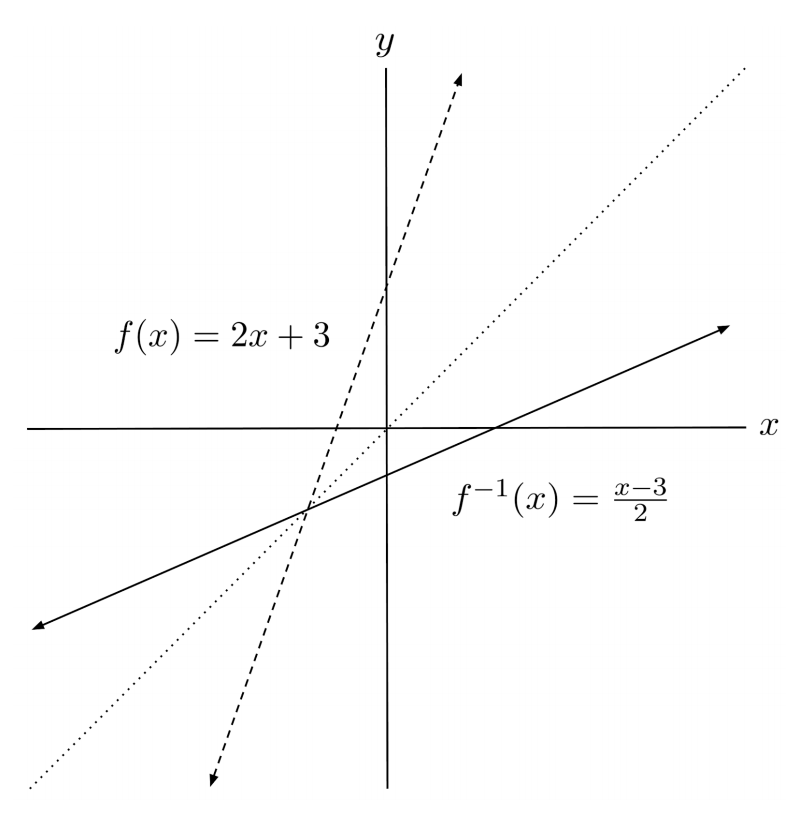## Case when No Inverse Exists

Graphically, we can see that some functions don’t have inverse functions. If reflecting the graph over the line $y=x$ causes multiple y-values to be associated with a single x-value, then this breaks the definition of a function, and the resulting graph is not a function.

Algebraically, an inverse function is supposed to take original outputs back to original inputs, but it can’t do this if it can’t distinguish which input x-value caused the output y-value.

For example, the function $f(x)=x^2$ has $f(2)=f(-2)=4$, so when a supposed inverse function takes an output of $4$, it will not know whether the output came from the input $2$ or $-2$. Therefore, no inverse function can be constructed for $f(x)=x^2$.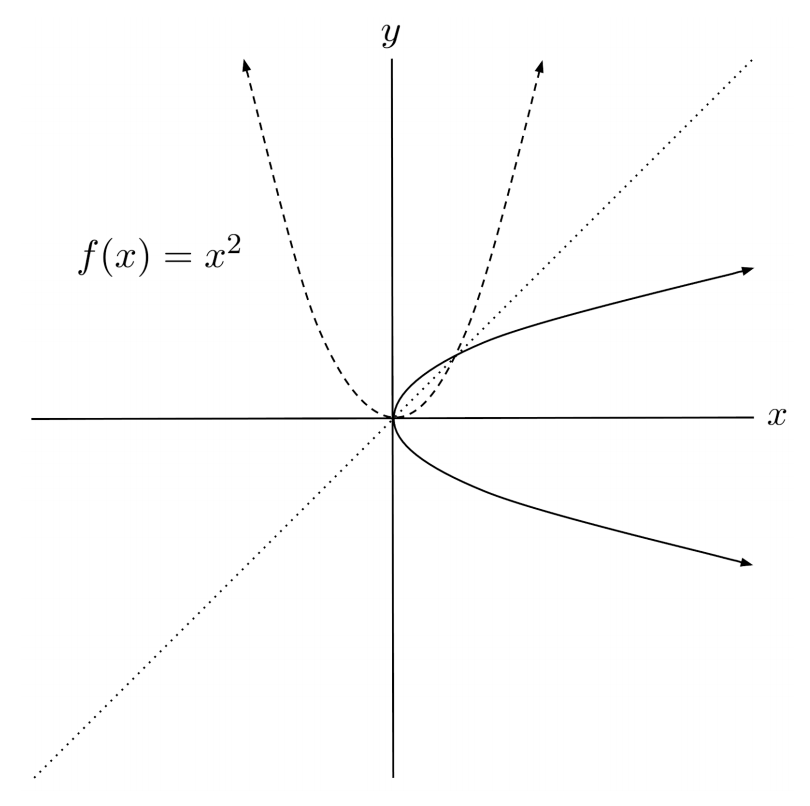## Domain Restrictions

That being said, inverse functions can be created if we restrict the domain, the set of allowed inputs.

For example, if we restrict the domain of $f(x)=x^2$ to only positive inputs, then the inverse function would know that an output of $4$ comes from an input of $2$.

We can also see this graphically – if we graph $f(x)=x^2$ only for positive values of $x$, then no x-value has multiple y-values when we reflect the graph over the line $y=x$.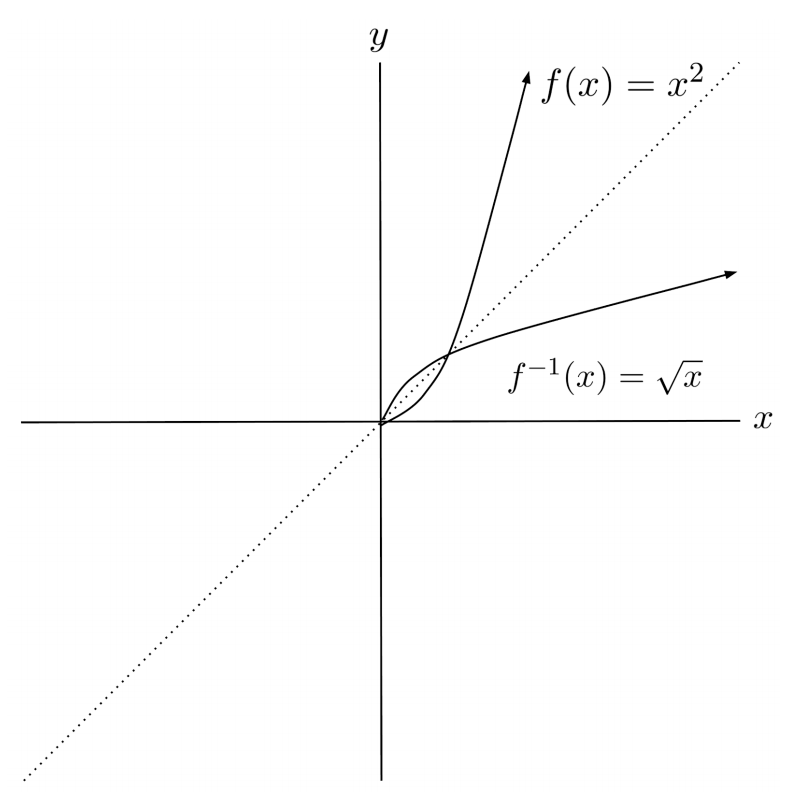## Exercises

Sketch the original function and the graph of the supposed inverse by reflecting the original function $f$ over the line $y=x$. Then, if the inverse function $f^{-1}$ exists, use algebra to find its equation. (You can view the solution by clicking on the problem.)

$1) \hspace{.5cm} f(x)= 2x$
Solution:
$f^{-1}(x) = \frac{1}{2}x$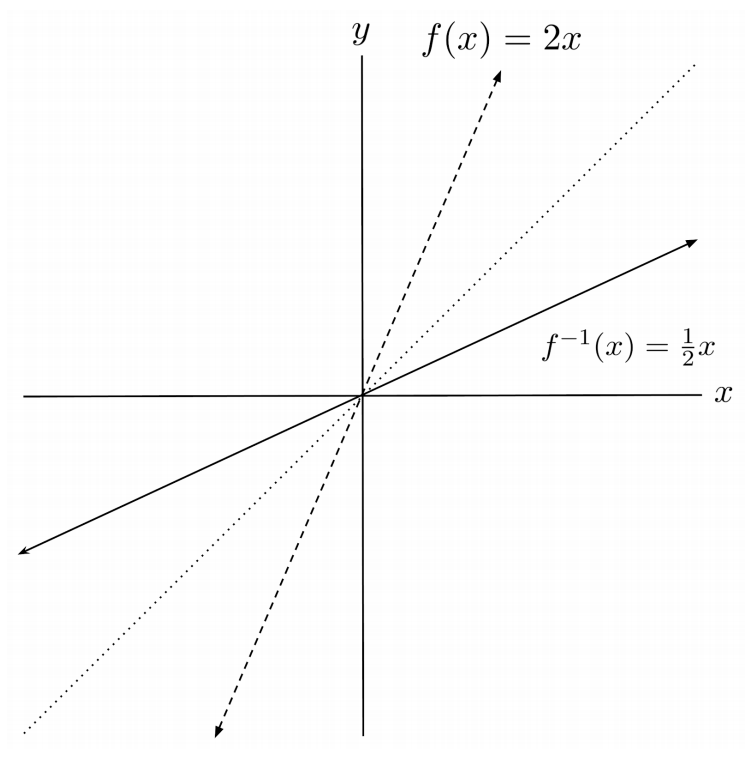$2) \hspace{.5cm} f(x)= 3x-3$
Solution:
$f^{-1}(x) = \frac{x+3}{3}$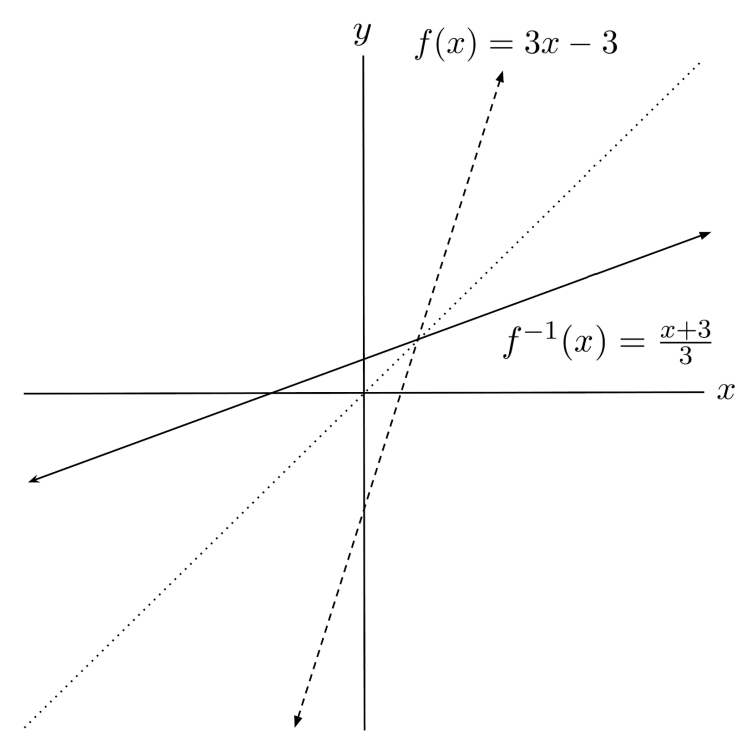$3) \hspace{.5cm} f(x)= \sqrt{2x}$
Solution:
$f^{-1}(x) = \frac{1}{2}x^2$
$\text{restriction: } x \geq 0$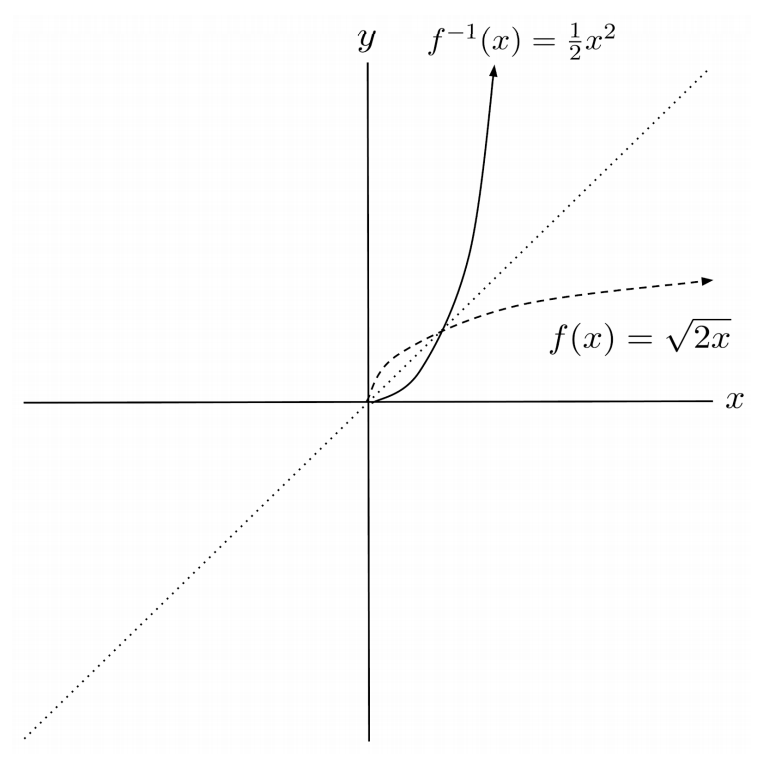$4) \hspace{.5cm} f(x)= x^2+1$
Solution:
$\text{no inverse function}$

$5) \hspace{.5cm} f(x)= x^3+1$
Solution:
$f^{-1}(x) = \sqrt{x-1}$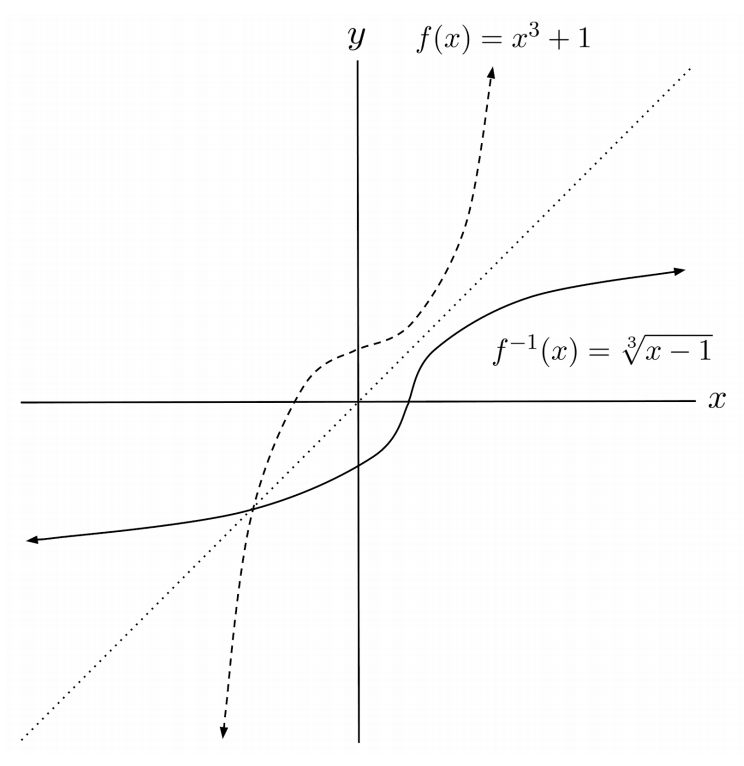$6) \hspace{.5cm} f(x)= |x|$
Solution:
$\text{no inverse function}$

$7) \hspace{.5cm} f(x)= -\frac{2}{x}$
Solution:
$f^{-1}(x) = -\frac{2}{x}$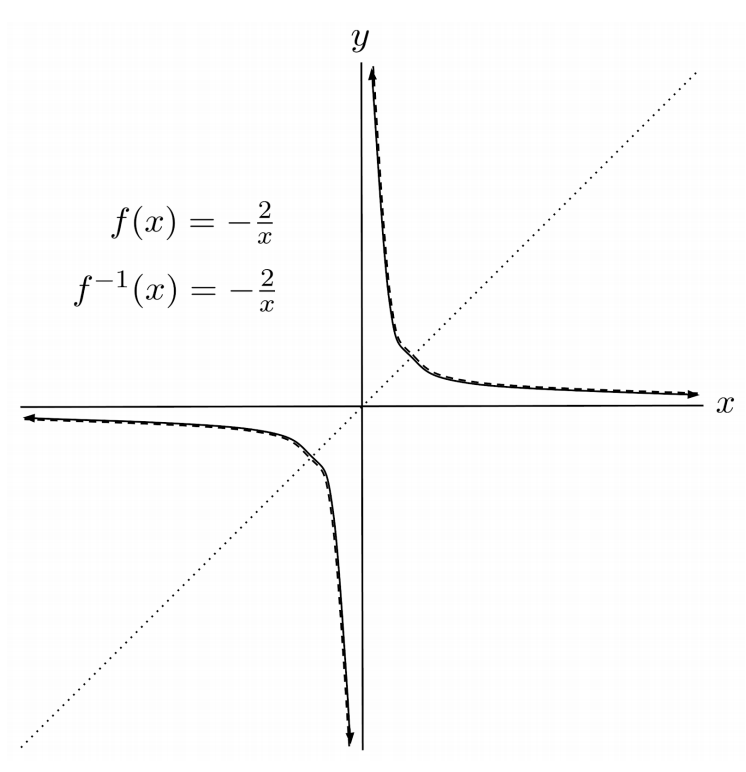$8) \hspace{.5cm} f(x)= \frac{1}{2x-1}$
Solution:
$f^{-1}(x) = \frac{1}{2x} + \frac{1}{2}$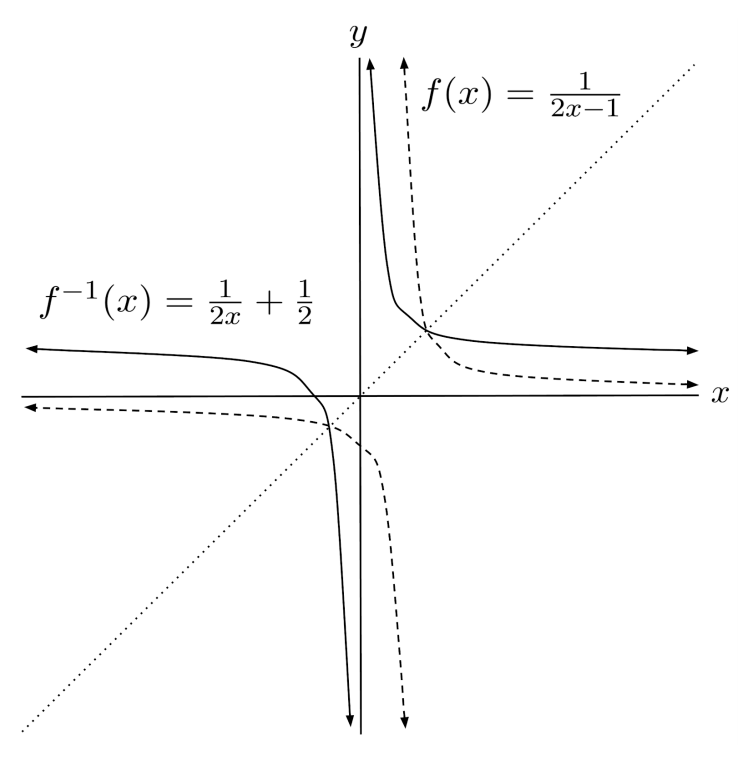$9) \hspace{.5cm} f(x)= \frac{1}{3x^2}$
Solution:
$\text{no inverse function}$

$10) \hspace{.5cm} f(x)= 3^x+4$
Solution:
$f^{-1}(x) = \log_3 (x-4)$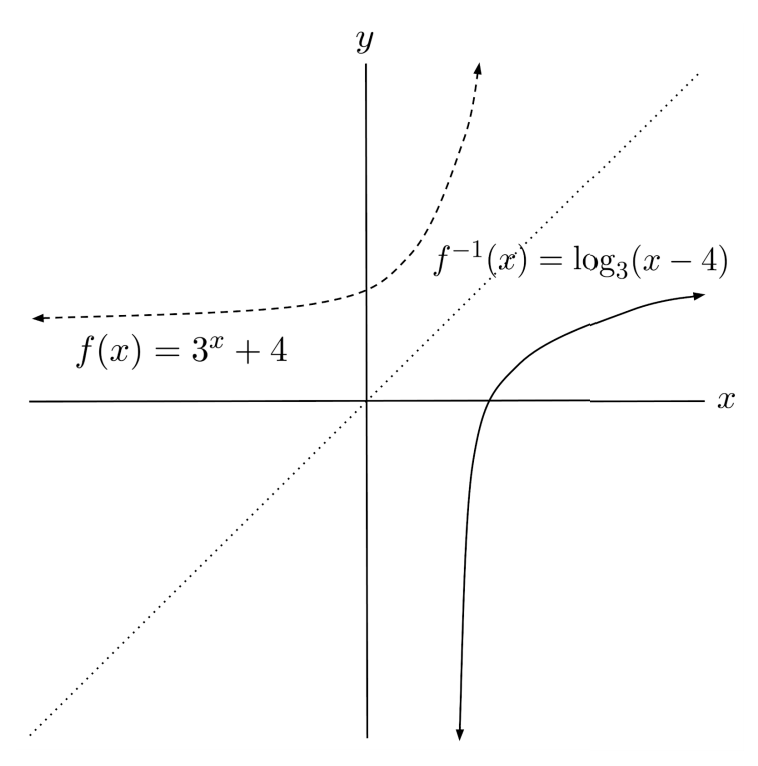$11) \hspace{.5cm} f(x)= \frac{3}{2} \log_2 (x+3)$
Solution:
$f^{-1}(x) = 2^{\frac{2}{3}x} - 3$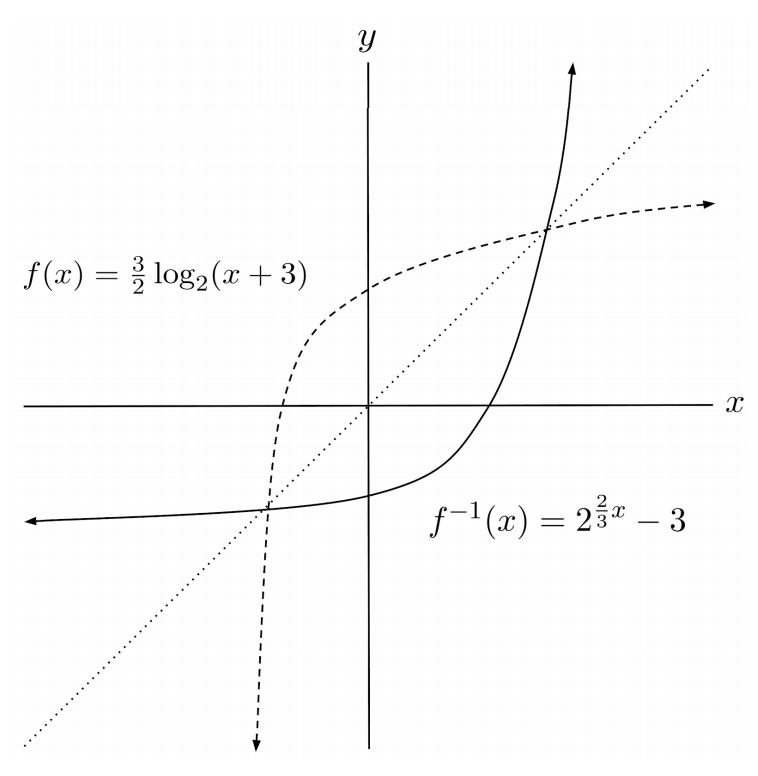$12) \hspace{.5cm} f(x)= 4$
Solution:
$\text{no inverse function}$

\begin{align*} 13) \hspace{.5cm} &f(x)= -3x^2 \\ &\text{restriction: } x>0 \end{align*}
Solution:
$f^{-1}(x) = \sqrt{ - \frac{1}{3}x }$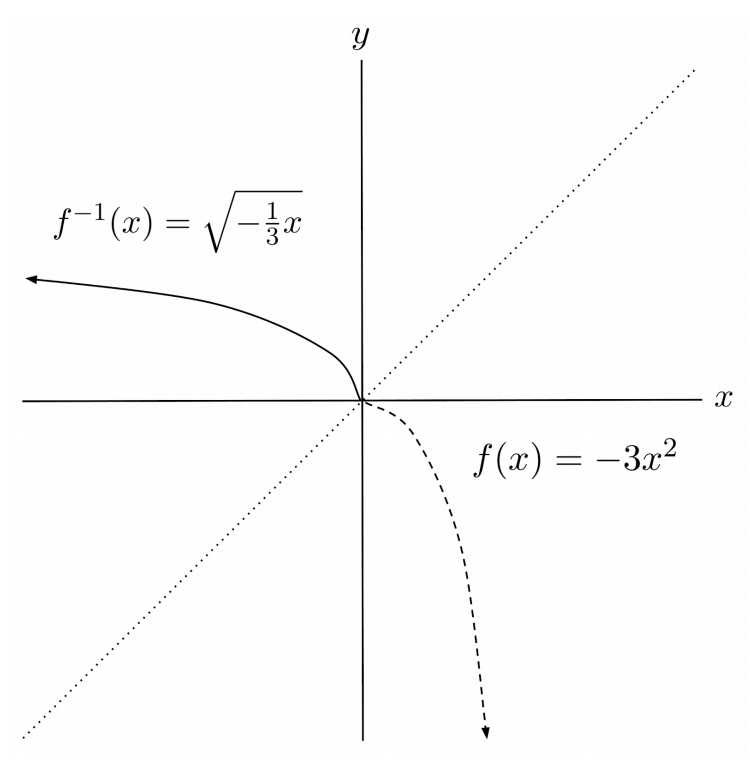\begin{align*} 14) \hspace{.5cm} &f(x)= \frac{1}{2}x^2 \\ &\text{restriction: } x<0 \end{align*}
Solution:
$f^{-1}(x) = -\sqrt{2x}$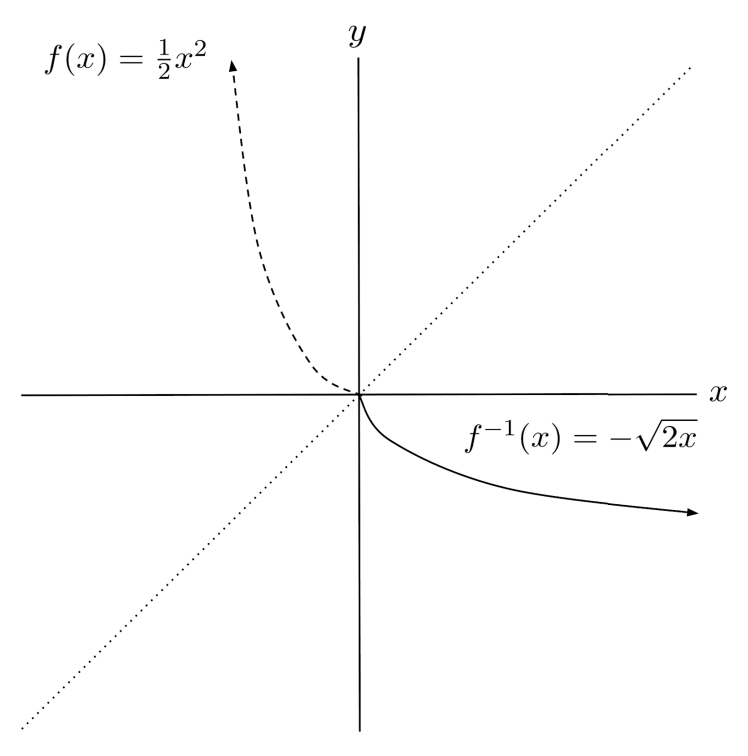\begin{align*} 15) \hspace{.5cm} &f(x)= |2x-3| \\ &\text{restriction: } x \geq \frac{3}{2} \end{align*}
Solution:
$f^{-1}(x) = \frac{x+3}{2}$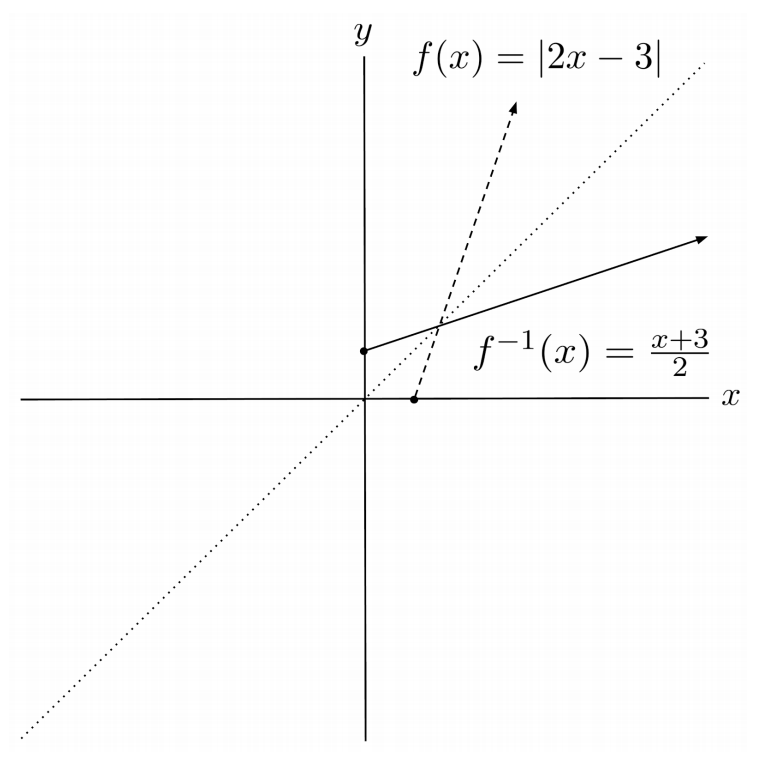\begin{align*} 16) \hspace{.5cm} &f(x)= -\left| 1-\frac{1}{3}x \right| \\ &\text{restriction: } x>3 \end{align*}
Solution:
$f^{-1}(x) = -3x+3$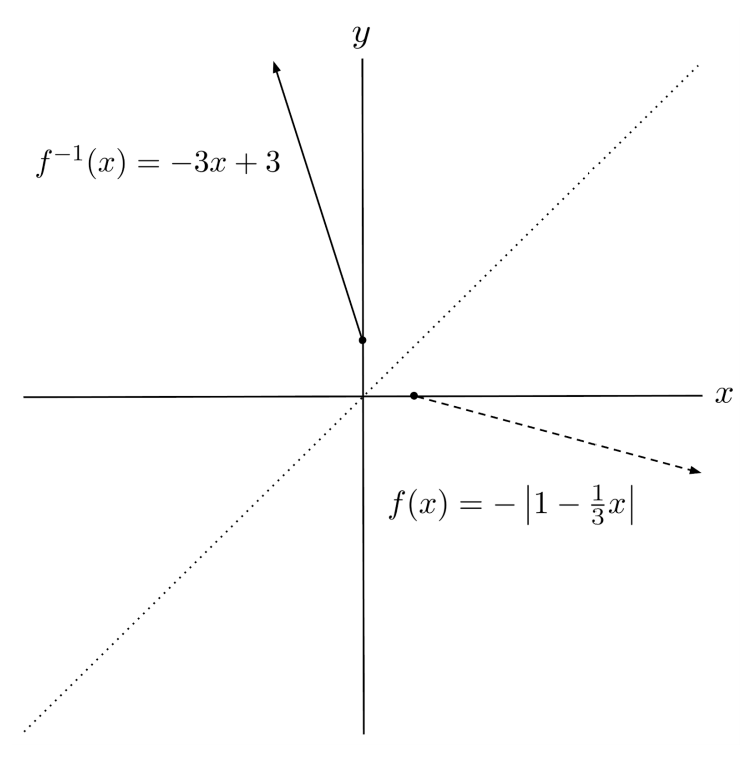This post is part of a series.